# Dice 7

A standard number cube is tossed 210 times. What is a reasonable prediction for the number of times the number cube will land on a 5?

n =  35

### Step-by-step explanation: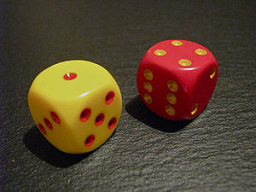Did you find an error or inaccuracy? Feel free to write us. Thank you!Tips to related online calculators
Would you like to compute count of combinations?

## Related math problems and questions:

• Dice and coinA number cube is rolled and a coin is tossed. The number cube and the coin are fair. What is the probability that the number rolled is greater than 2 and the coin toss is head?
• DiceWe throw 10 times a playing dice. What is the probability that the six will fall exactly 4 times?
• One dice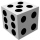Calculate the probability of one dice roll with the numbers 1, 2, 3, 4, 5, 6 on the walls. Write the results in a notebook in the shape of a fraction in the basic form: 2/3. a, The number 1 falls on the cube. b, The number 5 falls on the cube. c, An even
• Fall sum or sameFind the probability that if you roll two dice, it will fall the sum of 10, or the same number will fall on both dice.
• DiceWe throw five times the dice. What is the probability that six fits exactly twice?
• Perfect cubesSuppose a number is chosen at random from the set (0,1,2,3,. .. ,202). What is the probability that the number is a perfect cube?
• Roll the diceWhat is the probability that if we roll the dice, a number less than 5 falls?
• Sum 10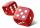What is the probability that two dice throw twice in a row will result the sum of 10?
• We rollWe roll two dice A. - what is the probability that the sum of the falling numbers is at most 4 B. - is at least 10 C. - is divisible by 5?
• Two diceWe roll two dice. What is the probability that the sum of the falling numbers is greater than 3?
• The diceWhat is the probability of events that if we throw a dice is rolled less than 6?
• Six on diceWhat is the probability that when throwing two dice will fall at least one six?
• Dice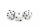How many times must throw the dice, the probability of throwing at least one six was greater than 90%?
• DicesWe will throw two dice. What is the probability that the ratio between numbers on first and second dice will be 1:2?
• Three dice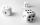When you throw three dice was the sum total of the dice 10. The yellow dice fell one eye more than on the red and brown fell 3 eyes less than red. How many eyes fell on every dice?
• Three dice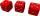What is the probability that when we roll three dice, we roll the numbers 1,2,3?
• One threeWe throw two dice. What is the probability that max one three falls?